# PSAT Math : How to find the height of a triangle

## Example Questions

### Example Question #1 : How To Find The Height Of A Triangle

A triangle has a base of 5 cm and an area of 15 cm. What is the height of the triangle?

None of the above

3 cm

5 cm

6 cm

1.5 cm

6 cm

Explanation:

The area of a triangle is (1/2)*base*height. We know that the area = 15 cm, and the base is 5 cm, so:

15 = 1/2 * 5 * height

3 = 1/2 * height

6 = height

### Example Question #2 : How To Find The Height Of A Triangle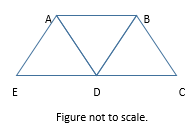In the figure above, AB = AD = AE = BD = BC = CD = DE = 1. What is the distance from A to C?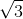Explanation: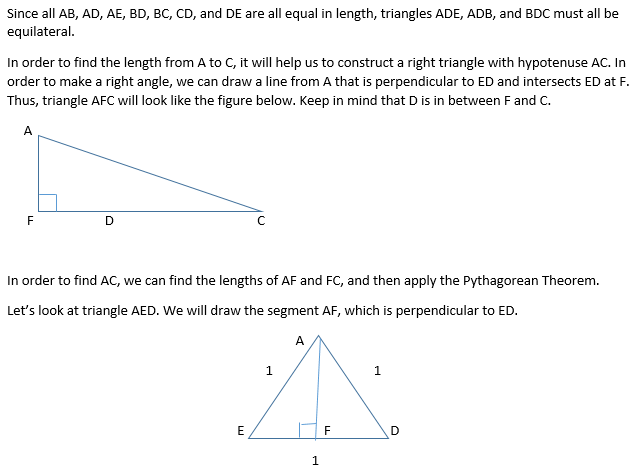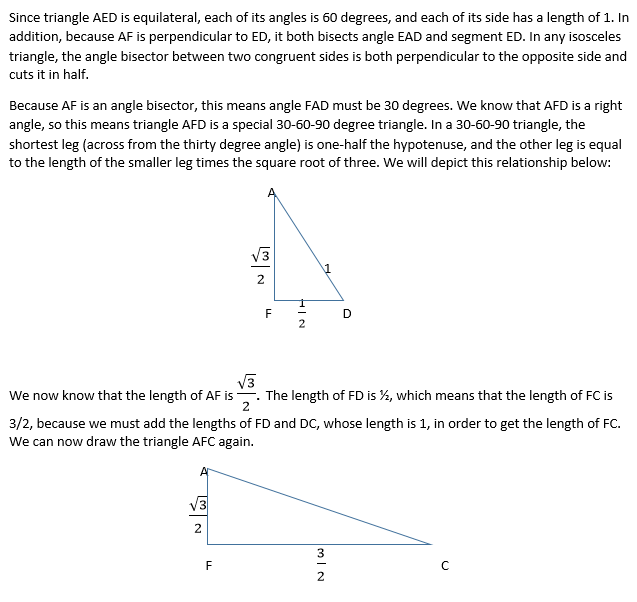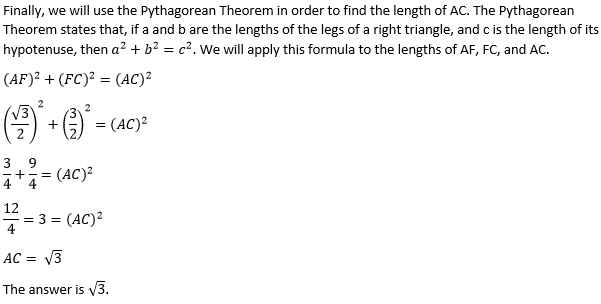### Example Question #1 : How To Find The Height Of A Triangle

A triangles has sides of 5, 9, and. Which of the folowing CANNOT be a possible value of?